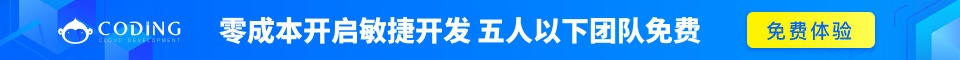PHP 5 数据类型

String（字符串）, Integer（整型）, Float（浮点型）, Boolean（布尔型）, Array（数组）, Object（对象）, NULL（空值）。

PHP 字符串

实例

<?php
\$x = "Hello world!";
echo \$x;
echo "<br>";
\$x = 'Hello world!';
echo \$x;
?>

PHP 整型

• 整数必须至少有一个数字 (0-9)
• 整数不能包含逗号或空格
• 整数是没有小数点的
• 整数可以是正数或负数
• 整型可以用三种格式来指定：十进制， 十六进制（ 以 0x 为前缀）或八进制（前缀为 0）。

实例

<?php
\$x = 5985;
var_dump(\$x);
echo "<br>";
\$x = -345; // negative number
var_dump(\$x);
echo "<br>";
\$x = 0x8C; // hexadecimal number
var_dump(\$x);
echo "<br>";
\$x = 047; // octal number
var_dump(\$x);
?>

<?php
\$x = 10.365;
var_dump(\$x);
echo "<br>";
\$x = 2.4e3;
var_dump(\$x);
echo "<br>";
\$x = 8E-5;
var_dump(\$x);
?>

\$x=true;
\$y=false;

PHP 数组

实例

<?php
\$cars=array("Volvo","BMW","Toyota");
var_dump(\$cars);
?>

PHP 对象

实例

<?php
class Car
{
var \$color;
function Car(\$color="green") {
\$this->color = \$color;
}
function what_color() {
return \$this->color;
}
}
?>

PHP NULL 值

NULL 值表示变量没有值。NULL 是数据类型为 NULL 的值。

NULL 值指明一个变量是否为空值。 同样可用于数据空值和NULL值的区别。

实例

<?php
\$x="Hello world!";
\$x=null;
var_dump(\$x);
?>